# Chapter 12 Electricity

Electricity is a form of energy that revolutionized the human lifestyle, today life is totally dependent on electric appliances. Electricity even has great advantages as its efficiency, easily transmitted, cleanness. Use of electricity is growing very fast with substitute of natural resources. In this chapter we shall study the basic concept of electricity, electric charge and its properties, conductor and insulator, electric potential, ohm's law, resistance and heating effect of current and its applications.

Download pdf of NCERT Examplar with Solutions for Class Science Chapter 12 Electricity

### Exercise 1

•  Q1 What does an electric circuit mean? Ans: An electric circuit is a closed  path of conducting wires through which electrons flow in  electric devices, switching devices, source of electricity, etc. Q2 Define the unit of current. Ans: The SI unit of electric current is Ampere. 1 ampere  is defined as the flow of 1 Coulomb  of charge through a wire in 1 second. Smaller unit  of  current  is milliAmpere that is 1/1000 ampere. Q3 Calculate the number of electrons constituting one coulomb of charge. Ans: Charge is flow of number of electrons. Q=ne Q is charge, n = number, e = electron Whereas, One electron possesses a charge of 1.6 × 10−19 C,  i.e., 1.6 × 10−19 C of charge is contained in 1 electron. ∴ 1 C of charge is contained in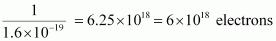Hence, 6x1018 electrons constitute one coulomb of charge.

### Exercise 2

•  Q1 Name a device that helps to maintain a potential difference across a conductor. Ans: Potential difference across a conductor is created by devices such as cell, battery, power supply, generator etc. Q2 What is meant by saying that the potential difference between two points is 1 V? Ans: Potential difference is defined as the work done to move per unit charge between the two ends of the current carrying conductor. Potential difference is designated by V.              V=W/Q If 1 J of work is required to move a charge of amount 1 C from one point to another, then it is said that the potential difference between the two points is 1 V. Q3 How much energy is given to each coulomb of charge passing through a 6 V battery? Ans: The amount of work done is equal to energy consumed. The energy given to each coulomb of charge is equal to the amount of work required to move unit charge Potential difference =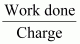Work Done = Potential Difference x Charge Given, Charge = 1 C Potential difference = 6 V Work Done = 6 x 1 = 6 J Total 6 J of energy is given to each coulomb of charge passing through a battery of 6 V.

### Exercise 3

•  Q1 On what factors does the resistance of a conductor depend? Ans: Resistance is the tendency of a resistor to oppose the flow of current. R= ρl/A R is resistance, ρ is resistivity, l is length of conductor and A is area of cross section of current carrying conductor. It depends upon the following factors: (a) Temperature of the conductor (b) Cross-sectional area of the conductor (c) Length of the conductor (d) Material of the conductor Q2 Will current flow more easily through a thick wire or a thin wire of the same material, when connected to the same source? Why? Ans: Resistance of a conductor is inversely proportional to the area of cross-section of the wire.Thicker the wire, lower is the resistance of the wire and vice-versa as current can flow more easily through a thick wire than a thin wire.  Resistance is tendency that oppose the flow of current which depends upon                       R=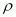l/A= Resistivity of the material of the wire l = Length of the wire A = Area of cross-section of the wire Q3 Let the resistance of an electrical component remains constant while the potential difference across the two ends of the component decreases to half of its former value. What change will occur in the current through it? Ans: According to ohm's law we know that current is directly potential to the potential difference across the two ends. V = IR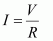Here, R= Resistance of the electrical component  V= Potential difference  and  I=Current  The potential difference is reduced by half, keeping resistance constant. Let the new resistance be R' and the new amount of current be I '. Therefore, from Ohm’s law, we obtain the amount of new current.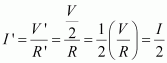So, the amount of current flowing through the electrical component is reduced by half. Q4 Why are coils of electric toasters and electric irons made of an alloy rather than a pure metal? Ans: An alloy has higher resistivity than the pure metal and offers more resistance. Moreover, at high temperatures, the alloys do not melt. Hence, the coils of heating appliances such as electric toasters and electric irons are made of an alloy rather than a pure metal. Q5 Use the data in Table 12.2 to answer the following – (a) Which among iron and mercury is a better conductor? (b) Which material is the best conductor? Ans: (a) The Resistivity of mercury is more than that of iron. So mercury offer more resistance than that iron whereas iron have lower resistivity and offer less resistance therefore iron is a better conductor than mercury Resistivity of iron = 10.0 x 10-8Resistivity of mercury = 94.0 × 10−8(b) Silver is the best conductor of electricity because of lowest resistivity and offers lower resistance as shown according to the above table.

### Exercise 4

•  Q1 Draw a schematic diagram of a circuit consisting of a battery of three cells of 2 V each, a 5 Ω resistor, an 8 Ω resistor, and a 12 Ω resistor, and a plug key, all connected in series. Ans: In a given circuit diagram  here shows three resistors of resistances 5 Ω, 8 Ω and 12 Ω respectively that are connected in series and a battery of potential 6 V.Three cells of potential 2 V, each connected in series, is equivalent to a battery of potential 2 V + 2 V + 2 V = 6V.Q2 Redraw the circuit of Question 1, putting in an ammeter to measure the current through the resistors and a voltmeter to measure the potential difference across the 12 Ω resistor. What would be the readings in the ammeter and the voltmeter? Ans: Current in a circuit is measured through an ammeter that  should be connected in the circuit in series with the resistors. To measure the potential difference across the 12 Ω resistor, a voltmeter should be connected parallel to this resistor, as shown in the following figure.The resistances are connected in series. Ohm’s law can be used to obtain the readings of ammeter and voltmeter. According to it  V = IR, Here  , V Potential difference= 6 V Current flowing through the circuit/resistors = I Resistance of the circuit, R = 5+8+12 = 25 ΩPotential difference across 12 Ω resistor = VI Current flowing through the 12 Ω resistor, I = 0.24 A Therefore, using Ohm’s law, we obtain VI = IR = 0.24x12 = 2.88 V So the reading of the ammeter will be 0.24 A. The reading of the voltmeter will be 2.88 V.

### Exercise 5

•  Q1 Judge the equivalent resistance when the following are connected in parallel – (a) 1 Ω and 106 Ω, (b) 1 Ω and 103 Ω, and 106 Ω. Ans: (a) When 1 Ω and 106 Ω are connected in parallel: Let R be the equivalent resistance.Therefore, equivalent resistance 1 Ω (b) When 1 Ω, and are connected in parallel: Let R be the equivalent resistance.Therefore, equivalent resistance = 0.999 Ω Q2 An electric lamp of 100 Ω, a toaster of resistance 50 Ω, and a water filter of resistance 500 Ω are connected in parallel to a 220 V source. What is the resistance of an electric iron connected to the same source that takes as much current as all three appliances, and what is the current through it? Ans: Resistance of electric lamp, R1 = 100 Ω Resistance of toaster, R2 = 50 Ω Resistance of water filter, R3 = 500 Ω Voltage of the source, V = 220 V These are connected in parallel, as shown in the following figure.Let R be the equivalent resistance of the circuit.According to Ohm’s law, V = IRWhere, Current flowing through the circuit = I7.04 A of current is drawn by all the three given appliances. Therefore, current drawn by an electric iron connected to the same source of potential 220 V = 7.04 A Let R' be the resistance of the electric iron. According to Ohm’s law,Therefore, the resistance of the electric iron is 31.25 Ω and the current flowing through it is 7.04 A. Q3 What are the advantages of connecting electrical devices in parallel with the battery instead of connecting them in series? Ans: There is no division of voltage among the appliances when connected in parallel. The potential difference across each appliance is equal to the supplied voltage. The total effective resistance of the circuit can be reduced by connecting electrical appliances in parallel. Q4 How can three resistors of resistances 2 Ω, 3 Ω, and 6 Ω be connected to give a total resistance of (a) 4 Ω, (b) 1 Ω? Ans: There are three resistors of resistances 2 Ω, 3 Ω, and 6 Ω respectively. (a) The following circuit diagram shows the connection of the three resistors.Here, 6 Ω and 3 Ω resistors are connected in parallel. Therefore, their equivalent resistance will be given byThis equivalent resistor of resistance 2 Ω is connected to a 2 Ω resistor in series. Therefore, equivalent resistance of the circuit = 2 Ω + 2 Ω = 4 Ω Hence, the total resistance of the circuit is 4 Ω. (b) The following circuit diagram shows the connection of the three resistors.All the resistors are connected in series. Therefore, their equivalent resistance will be given asTherefore, the total resistance of the circuit is 1 Ω. Q5 What is (a) the highest, (b) the lowest total resistance that can be secured by combinations of four coils of resistance 4 Ω, 8 Ω, 12 Ω, 24 Ω? Ans: There are four coils of resistances 4 Ω, 8 Ω, 12 Ω, and 24 Ω respectively. (a) If these coils are connected in series, then the equivalent resistance will be the highest, given by the sum 4 + 8 + 12 + 24 = 48 Ω (b) If these coils are connected in parallel, then the equivalent resistance will be the lowest, given byTherefore, 2 Ω is the lowest total resistance.

### Exercise 6

•  Q1 Why does the cord of an electric heater not glow while the heating element does? Ans: The heating element of an electric heater is a resistor. The amount of heat produced by it is proportional to its resistance. The resistance of the element of an electric heater is very high. As current flows through the heating element, it becomes too hot and glows red. On the other hand, the resistance of the cord is low. It does not become red when current flows through it. Q2 Compute the heat generated while transferring 96000 coulomb of charge in one hour through a potential difference of 50 V. Ans: The amount of heat (H) produced is given by the Joule’s law of heating as H = VIt Where, Voltage, V = 50 V Time, t = 1 h = 1 × 60 × 60 s Amount of current,Therefore, the heat generated is 4.8 x 106 J. Q3 An electric iron of resistance 20 Ω takes a current of 5 A. Calculate the heat developed in 30 s. Ans: The amount of heat (H) produced is given by the joule’s law of heating as H = VIt Where, Current, I = 5 A Time, t = 30 s Voltage, V = Current × Resistance = 5 × 20 = 100 V H = 100 x 5 x 30 = 1.5x104 J Therefore, the amount of heat developed in the electric iron is 1.5x104 J.

### Exercise 7

•  Q1 What determines the rate at which energy is delivered by a current? Ans: The rate of consumption of electric energy in an electric appliance is called electric power. Hence, the rate at which energy is delivered by a current is the power of the appliance. Q2 An electric motor takes 5 A from a 220 V line. Determine the power of the motor and the energy consumed in 2 h. Ans: Power (P) is given by the expression, P = VI Where, Voltage, V = 220 V Current, I = 5 A P = 220 x 5 = 1100 W Energy consumed by the motor = Pt Where, Time, t = 2 h = 2 × 60 × 60 = 7200 sP = 1100 × 7200 = 7.92 × 106 J Therefore, power of the motor = 1100 W Energy consumed by the motor = 7.92 × 106 J

### Exercise 8

•  Q1 A piece of wire of resistance R is cut into five equal parts. These parts are then connected in parallel. If the equivalent resistance of this combination is R′, then the ratio R/R′ is – (a) 1/25        (b) 1/5        (c) 5        (d) 25 Ans: (d) Resistance of a piece of wire is proportional to its length. A piece of wire has a resistance R. The wire is cut into five equal parts. Therefore, resistance of each part =All the five parts are connected in parallel. Hence, equivalent resistance (R’) is given asTherefore, the ratiois 25. Q2 Which of the following terms does not represent electrical power in a circuit? (a) I2R          (b) IR2          (c) VI          (d) V2/R Ans: (b) Electrical power is given by the expression, P = VI … (i) According to Ohm’s law, V = IR … (ii) Where, V = Potential difference I = Current R = ResistanceP = VI From equation (i), it can be written P = (IR) × IP = I2R From equation (ii), it can be writtenPower P cannot be expressed as IR2. Q3 An electric bulb is rated 220 V and 100 W. When it is operated on 110 V, the power consumed will be – (a) 100 W          (b) 75 W          (c) 50 W          (d) 25 W Ans: (d) Energy consumed by an appliance is given by the expression,Where, Power rating, P = 100 W Voltage, V = 220 VThe resistance of the bulb remains constant if the supply voltage is reduced to 110 V. If the bulb is operated on 110 V, then the energy consumed by it is given by the expression for power asTherefore, the power consumed will be 25 W. Q4 Two conducting wires of the same material and of equal lengths and equal diameters are first connected in series and then parallel in a circuit across the same potential difference. The ratio of heat produced in series and parallel combinations would be – (a) 1:2          (b) 2:1          (c) 1:4          (d) 4:1 Ans: (c) The Joule heating is given by, H = i2Rt Let, R be the resistance of the two wires. The equivalent resistance of the series connection is RS = R + R = 2R If V is the applied potential difference, then it is the voltage across the equivalent resistance.The heat dissipated in time t is,The equivalent resistance of the parallel connection isV is the applied potential difference across this RP.The heat dissipated in time t is,So, the ratio of heat produced is,Note: Halso Hi2 and Ht. In this question, t is same for both the circuit. But the current through the equivalent resistance of both the circuit is different. We could have solved the question directly using HR if in case the current was also same. As we know the voltage and resistance of the circuits, we have calculated i in terms of voltage and resistance and used in the equation H = i2Rt to find the ratio. Q5 How is a voltmeter connected in the circuit to measure the potential difference between two points? Ans: To measure the potential difference between two points, a voltmeter should be connected in parallel to the points. Q6 A copper wire has diameter 0.5 mm and resistivity of 1.6 × 10–8 Ω m. What will be the length of this wire to make its resistance 10 Ω? How much does the resistance change if the diameter is doubled? Ans: Resistance (R) of a copper wire of length l and cross-section A is given by the expression,Where, Resistivity of copper, p = 1.6 x 10-8 Ω m Area of cross-section of the wire,Diameter = 0.5 mm = 0.0005 m Resistance, R = 10 Ω Hence, length of the wire,If the diameter of the wire is doubled, new diameter = 2x0.5 = 1 mm = 0.001 m Therefore, resistance R'Therefore, the length of the wire is 122.7 m and the new resistance is 2.5 Ω Q7 The values of current I flowing in a given resistor for the corresponding values of potential difference V across the resistor are given below – I (amperes)   0.5   1.0   2.0   3.0    4.0 V (volts)        1.6   3.4   6.7   10.2  13.2 Plot a graph between V and I and calculate the resistance of that resistor. Ans: The plot between voltage and current is called IV characteristic. The voltage is plotted on x-axis and current is plotted on y-axis. The values of the current for different values of the voltage are shown in the given table. V (volts)        1.6    3.4    6.7    10.2   13.2 I (amperes)   0.5    1.0    2.0    3.0     4.0 The IV characteristic of the given resistor is plotted in the following figure.The slope of the line gives the value of resistance (R) as,Therefore, the resistance of the resistor is 3.4 Ω. Q8 When a 12 V battery is connected across an unknown resistor, there is a current of 2.5 mA in the circuit. Find the value of the resistance of the resistor. Ans: Resistance (R) of a resistor is given by Ohm’s law as,Where, Potential difference, V = 12 V Current in the circuit, I = 2.5 mA = 2.5x10-3 ATherefore, the resistance of the resistor is 4.8 kΩ. Q9 A battery of 9 V is connected in series with resistors of 0.2 Ω, 0.3 Ω, 0.4 Ω , 0.5 Ω and 12 Ω, respectively. How much current would flow through the 12 Ω resistor? Ans: There is no current division occurring in a series circuit. Current flow through the component is the same, given by Ohm’s law asWhere, R is the equivalent resistance of resistances 0.2 Ω, 0.3 Ω, 0.4 Ω, 0.5 Ω and 12 Ω. These are connected in series. Hence, the sum of the resistances will give the value of R. R = 0.2 + 0.3 + 0.4 + 0.5 + 12 = 13.4 Ω Potential difference, V = 9 VTherefore, the current that would flow through the 12 Ω resistor is 0.671 A. Q10 How many 176 Ω resistors (in parallel) are required to carry 5 A on a 220 V line? Ans: For x number of resistors of resistance 176 Ω, the equivalent resistance of the resistors connected in parallel is given by Ohm’s law asWhere, Supply voltage, V = 220 V Current, I = 5 A Equivalent resistance of the combination = R, given asFrom Ohm’s law,Therefore, four resistors of 176 Ω are required to draw the given amount of current. Q11 Show how you would connect three resistors, each of resistance 6 Ω, so that the combination has a resistance of (i) 9 Ω, (ii) 4 Ω. Ans: If we connect the resistors in series, then the equivalent resistance will be the sum of the resistors, i.e., 6 Ω + 6 Ω + 6 Ω = 18 Ω, which is not desired. If we connect the resistors in parallel, then the equivalent resistance will bewhich is also not desired. Hence, we should either connect the two resistors in series or parallel. (i) Two resistors in parallelTwo 6 Ω resistors are connected in parallel. Their equivalent resistance will beThe third 6 Ω resistor is in series with 3 Ω. Hence, the equivalent resistance of the circuit is 6 Ω + 3 Ω = 9 Ω. (ii) Two resistors in seriesTwo 6 Ω resistors are in series. Their equivalent resistance will be the sum 6 + 6 = 12 Ω The third 6 Ω resistor is in parallel with 12 Ω. Hence, equivalent resistance will beTherefore, the total resistance is 4 Ω. Q12 Several electric bulbs designed to be used on a 220 V electric supply line, are rated 10 W. How many lamps can be connected in parallel with each other across the two wires of 220 V line if the maximum allowable current is 5 A? Ans: Resistance R1 of the bulb is given by the expression,Where, Supply voltage, V = 220 V Maximum allowable current, I = 5 A Rating of an electric bulb P1 = 10 WAccording to Ohm’s law, V = I R Where, R is the total resistance of the circuit for x number of electric bulbsResistance of each electric bulb, R1 = 4840 ΩTherefore, 110 electric bulbs are connected in parallel. Q13 A hot plate of an electric oven connected to a 220 V line has two resistance coils A and B, each of 24 Ω resistance, which may be used separately, in series, or in parallel. What are the currents in the three cases? Ans: Supply voltage, V = 220 V Resistance of one coil, R = 24 Ω (i) Coils are used separately According to Ohm’s law, V = I1R1 Where, I1 is the current flowing through the coilTherefore, 9.16 A current will flow through the coil when used separately. (ii) Coils are connected in series Total resistance, R2 = 24 Ω + 24 Ω = 48 Ω According to Ohm’s law, V = I2R2 Where, I2 is the current flowing through the series circuitTherefore, 4.58 A current will flow through the circuit when the coils are connected in series. (iii) Coils are connected in parallel Total resistance, R3 is given asAccording to Ohm’s law, V = I3R3 Where, I3 is the current flowing through the circuitTherefore, 18.33 A current will flow through the circuit when coils are connected in parallel. Q14 Compare the power used in the 2 Ω resistor in each of the following circuits: (i) a 6 V battery in series with 1 Ω and 2 Ω resistors, and (ii) a 4 V battery in parallel with 12 Ω and 2 Ω resistors. Ans: (i) Potential difference, V = 6 V 1 Ω and 2 Ω resistors are connected in series. Therefore, equivalent resistance of the circuit, R = 1 + 2 = 3 Ω According to Ohm’s law, V = IR Where, I is the current through the circuitThis current will flow through each component of the circuit because there is no division of current in series circuits. Hence, current flowing through the 2 Ω resistor is 2 A. Power is given by the expression, P = (I)2R = (2)2 x 2 = 8 W (ii) Potential difference, V = 4 V 12 Ω and 2 Ω resistors are connected in parallel. The voltage across each component of a parallel circuit remains the same. Hence, the voltage across 2 Ω resistor will be 4 V. Power consumed by 2 Ω resistor is given byTherefore, the power used by 2 Ω resistor is 8 W. Q15 Two lamps, one rated 100 W at 220 V, and the other 60 W at 220 V, are connected in parallel to electric mains supply. What current is drawn from the line if the supply voltage is 220 V? Ans: Both the bulbs are connected in parallel. Therefore, potential difference across each of them will be 220 V, because no division of voltage occurs in a parallel circuit. Current drawn by the bulb of rating 100 W is given by,Similarly, current drawn by the bulb of rating 60 W is given by,Q16 Which uses more energy, a 250 W TV set in 1 hr, or a 1200 W toaster in 10 minutes? Ans: Energy consumed by an electrical appliance is given by the expression, H = Pt Where, Power of the appliance = P Time = t Energy consumed by a TV set of power 250 W in 1 h = 250 × 3600 = 9 × 105 J Energy consumed by a toaster of power 1200 W in 10 minutes = 1200 × 600 = 7.2× 105 J Therefore, the energy consumed by a 250 W TV set in 1 h is more than the energy consumed by a toaster of power 1200 W in 10 minutes. Q17 An electric heater of resistance 8 Ω draws 15 A from the service mains 2 hours. Calculate the rate at which heat is developed in the heater. Ans: Rate of heat produced by a device is given by the expression for power as P = I2R Where, Resistance of the electric heater, R = 8 Ω Current drawn, I = 15 A P = (15)2 x 8 = 1800 J/s Therefore, heat is produced by the heater at the rate of 1800 J/s. Q18 Explain the following. (a) Why is the tungsten used almost exclusively for filament of electric lamps? (b) Why are the conductors of electric heating devices, such as bread-toasters and electric irons, made of an alloy rather than a pure metal? (c) Why is the series arrangement not used for domestic circuits? (d) How does the resistance of a wire vary with its area of cross-section? (e) Why are copper and aluminium wires usually employed for electricity transmission? Ans: (a) The melting point and resistivity of tungsten are very high. It does not burn readily at a high temperature. The electric lamps glow at very high temperatures. Hence, tungsten is mainly used as heating element of electric bulbs. (b) The conductors of electric heating devices such as bread toasters and electric irons are made of alloy because resistivity of an alloy is more than that of metals. It produces large amount of heat. (c) There is voltage division in series circuits. Each component of a series circuit receives a small voltage for a large supply voltage. As a result, the amount of current decreases and the device becomes hot. Hence, series arrangement is not used in domestic circuits. (d) Resistance (R) of a wire is inversely proportional to its area of cross-section (A), i.e.,(e) Copper and aluminium wires have low resistivity. They are good conductors of electricity. Hence, they are usually employed for electricity transmission.